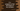# C++ tutorial to find the largest of two user input numbers# Introduction :

In this CPP tutorial, we will learn how to find the largest of two numbers programmatically. The program will ask the user to enter the numbers one by one.

With this program, you will learn how to read user inputs in C++, how to print a message to the user, how to store values in variables and how to use if-else condition.

## Algorithm to use :

Following is the algorithm we are using in this program :

1. Read the user input numbers one by one.

2. Compare them using an if-else condition.

3. Print the final output.

That’s it. Now let’s write these steps in code :

## C++ program :

``````#include <iostream>
using namespace std;

//1
int main()
{
//2
int firstNum;
int secondNum;

//3
cout << "Enter the first number : "; cin >> firstNum;

//4
cout << "Enter the second number : "; cin >> secondNum;

//5
if (firstNum > secondNum)
{
cout << firstNum << " is greater than " << secondNum<<endl;
}
else
{
cout << secondNum << " is greater than " << firstNum<<endl;
}
return 0;
}
``````

### Explanation :

The commented numbers in the above program denote the step numbers below :

1. main() is the first method that executes at the start of the program. All lines in this method will be executed one by one from top to bottom.

2. Inside this main() method, we are declaring two integer variables firstNum and secondNum. These variables will hold the first and second number.

3. Ask the user to enter the first number. Read this number and store it in the firstNum variable.

4. Similarly, ask the user to enter the second number. Read it and store it in the secondNum variable. We are using cout to print a message to the user and cin to read a value to an integer.

5. Using an if-else condition, check which number is greater. If the condition is written inside the if statement is true, it will execute the code inside the if statement. Else, it will execute the code in the else statement.

We are using cout to print the message.

### Examples :

``````Enter the first number : 12
Enter the second number : 22
22 is greater than 12

Enter the first number : 23
Enter the second number : 12
23 is greater than 12
``````

### Conclusion :

You can change this program to check for positive, negative numbers. if-else is one of the most used expressions in any programming language. In a real-world project, you will face these statements more often. Try to run the above program and drop one comment below if you have any questions.